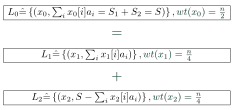## Tuesday, July 12, 2016

### The Subset-Sum Problem

The Subset-Sum problem (also known as knapsack problem) we want to review in this blog-post is defined as follows: Given $n, S, a_1, a_2, \dots a_n \in \mathbb{N}$, find \begin{align} I \subseteq [n] = \{1,2, \dots, n\}: \sum_{i \in I} a_i = S. \quad (1) \label{s} \end{align}
Historical Remarks

This old problem was first studied in 1897, the same year the first airborne mission to completely reach the geographical north pole (NP) started (and ended...), and was one of the first proven to be NP-complete -- worst-case instances of this problem are computationally intractable. Subset-Sum was proved to be NP-complete by reducing '3-SAT' to the 'Graph Coloring Problem' which was reduced to 'Exact cover' which was reduced to Knapsack and close variants thereof. These proofs were carried out during the early 1970's rigorous reduction proofs and Subset-Sum problem is featured on Karp's somewhat famous list of 21 NP-complete problems, all infeasible to solve on current computers & algorithms thus a possible basis for cryptographic primitives. In the following table one can see how the expected time/space requirements of algorithms solving (1) in hard cases evolved as the techniques were refined by modern research:Expected time and space requirements of algorithms solving (1) in average hard instances.
The time and space requirements of current approaches are considerably less than for performing exhaustive search, a generic meet in the middle attack or even a finer combinatorial split into four (or multiple) lists. The currently best known algorithm, asymptotically speaking, is a quantum algorithm. The problem of determining a lower bound of the run-time is still an open research question. It seems more difficult than to see a possible link between the declining polar bear population in the arctic regions and the retreating sea ice accelerated by the observable rapid climate change.

Let us review two classical techniques that led to remarkable speed-ups:

Technique 1 - Meet in the MiddleSchröppel-Shamir: Combining disjoint sub-problems of smaller weight.
Hard instances of the Subset-Sum problem are characterized by relatively large elements $(\log_2 a_i \approx n)$ and a balanced solution, i.e. $|I| \approx \frac n 2$ in Equation (1). Identifying subsets of $[n]$ with length $n$ vectors $x$ over the 'number-set' $\{0,1\}$ via $i \in I \Leftrightarrow x[i] = 1$ one constructs lists $L_1, L_2$ of pairs merged to a solution in $L_0$:
Algorithms based on the birthday-paradox construct expected collisions in the second component of the sub-problems in the lists $L_1, L_2$ forcing any $x \in L_0$ to fulfill (1). The difficulty is to estimate the list-size needed to observe the existence of one solution with high probability. It is desirable to ensure that it is more likely to terminate the algorithm with a non-empty $|L_0| \geq 1$ (i.e. have a solution) than the chance to see a polar bear towards the north-east or meet one in the middle of Svalbard, Norway.

Technique 2 - Enlarge Number SetBCJ11: Adding length $n$ sub-solutions increases the number-set.
The idea in Howgrave-Graham-Joux (2010) that was later extended by Becker-Coron-Joux (2011) was to allow multiple representations. This comes at the price of enlarging the number-set, i.e. having $$x_0[i] = x_1[i] + x_2[i] \not \in \{0, 1\}.$$ Additionally to the first improvements due to constructing colliding sums, constructing too many potential solutions and introducing a non-trivial filtering step to remove 'inconsistent' ones when merging the two lists $L_1$ and $L_2$ back into one, still gave an overall speed-up asymptotically.
The number-set used by the authors was $\{-1,0,1\}$, indicating a summand appearing on both sides of Equation (1).
After constructing sufficiently many sub-problems and their respective partial solutions a collision can be expected thus the combination forms a solution for the given instance.

Applications
The cryptanalytic methods for structurally approaching the Subset-Sum problem are valuable algorithmic meta-techniques also applicable to other NP-complete problems like lattice- or code-based problems.Credits: http://fav.me/d3a1n08
Such problems are promising candidates for the construction of post-quantum cryptosystems, cloud security applications and encrypted Polar Bear TV broadcasts. There is a conference focusing on such topics (Polar bears and NP-complete problems) coming up - stay tuned.

PS: The bad image quality, is due to blogger wouldn't let me include vector-graphics like .pdf or .eps nor directly render them giving latex code... :-(